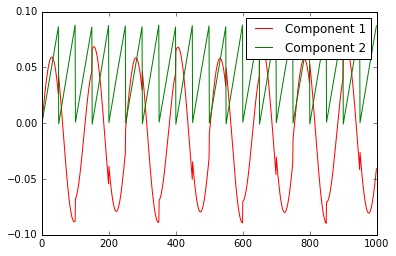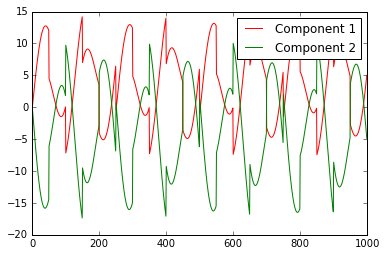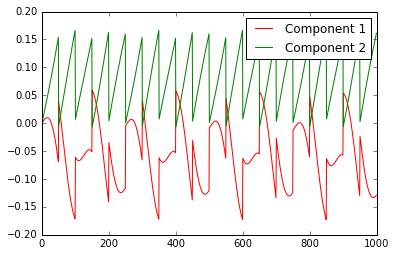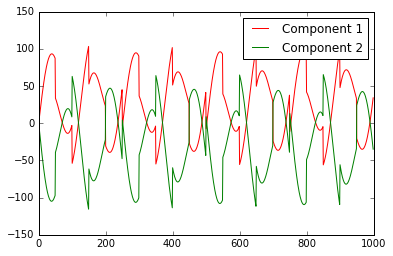线性独立成分分析(ICA)与鸡尾酒会问题 原##问题分析 设原始信号为S1、S2、...、Sn，一定存在一个mn的权重矩阵A，使得每个声音接收器对应A中一行。如m=n=2时，令A=[[0.6, 0.4], [0.45, 0.55]]，则第一个麦克风接收到的信号为x1=0.6S1+0.4S2，第二个麦克风接收到的信号为x2=0.45S1+0.55S2。即$$AS=x$$ 将该公式左右同乘以A的逆矩阵$$W=A^{-1}$$，得$$A^{-1}AS=A^{-1}x$$，即$$S=Wx$$，这样问题就解决了（当m≠n时，A不是方阵，此时可以乘A的伪逆）。于是问题来了：如何求A的逆矩阵W？

##独立成分分析(Independent Component Analysis) ICA算法始于Bell和Sejnowski，下面用极大似然估计简单推导一下ICA的求解过程：

###引理：已知x的概率分布$$f_{x}(x)$$，若$$Y=Ax$$，则对其累积分布函数有$$F_{Y}(y)=P{Y\leq y}=P{Ax\leq y}=P{x\leq y/A}=F_{X}(y/A)$$。求导得$$f_{Y}(y)=frac{f_{X}(x/A)}{|A|}$$。

def sigmoid(x):
return 1.0 / (1 + np.exp(-x))

def ica(x):
# x = np.array(x)
n = len(x)
m = len(x)
w = np.eye(n)
iw = np.zeros([n, n])
w1 = np.zeros([n, n])
alpha = 0.001

for time in range(200):
for i in range(m):
for j in range(n):
# 使用sigmoid函数作为CDF
t = 1 - 2 * sigmoid(np.dot(w[j], x[i]))
w1[j] = t * x[i]
# 使用arctan函数作为CDF
# t = -2 * np.dot(w[j], x[i]) / (1 + np.dot(w[j] ** 2, x[i] ** 2))
# w1[j] = t * x[i]
# 使用tanh函数作为CDF
# t = -2 * x[i] * np.tanh(np.dot(w[j], x[i]))
# w1[j] = t
# 使用erf函数(正态分布)的CDF
# t = -2 * np.dot(w[j], x[i])
# w1[j] = t * x[i]
iw = np.linalg.inv(w.transpose())
w1 += iw * alpha
w1 = w1.transpose() * alpha
w += w1
# print time, ":\t"
# print w
return w

##仿真结果 首先，使用python模拟，生成一个扫描波、一个正弦波如下；使用权重矩阵$$A=\begin{pmatrix} 0.6 & 0.4 \ 0.45 & 0.55 \end{pmatrix}\$$对原始信号进行加权，得到的混合信号如下：

import numpy as np
from matplotlib import pyplot as plt

def show(s1, s2, label1, label2):
x = [i for i in range(1000)]
plt.plot(x, s1, 'r', label = label1)
plt.hold(True)
plt.plot(x, s2, 'g', label = label2)
plt.hold()
plt.legend()
plt.show()

if __name__ == "__main__":
s1 = np.array([np.sin(float(x) / 20) for x in range(1000)])
s2 = np.array([float(x) / 50 for x in range(50)] * 20)
show(s1, s2, 'Signal 1', 'Signal 2')

A = np.array([[0.6, 0.4], [0.45, 0.55]])
x = np.dot(A, np.array([s1, s2])).transpose()
show(x[:,0], x[:,1], 'Mic 1', 'Mic 2')
# x -= x.mean()
w = ica(x)
[ps1, ps2] = np.dot(x, w).transpose()
show(ps1, ps2, 'Component 1', 'Component 2')不高不富不帅的陈政_

2018/12/27
0
0

2017/09/03
0
0

xinzhangyanxiang
2014/07/22
0
0

sm9sun
2017/12/09
0
0

aliceyangxi1987
2017/05/02
0
0

code-ortaerc

5
0

[TOC] 1.1.1标签操作v-text&v-html v-text：会把data中绑定的数据值原样输出。 v-html：会把data中值输出，且会自动解析html代码 <!--可以将指定的内容显示到标签体中--><标签 v-text=""></......

8
0
VMware vSphere的两种RDM磁盘

12
0
【AngularJS学习笔记】02 小杂烩及学习总结

16
0
Linux 内核的五大创新

20
0